Lecture on Zodiac Signs is Here

There is really huge diversity in the Universe...

There are stars...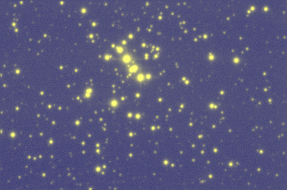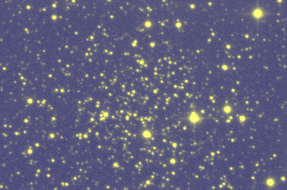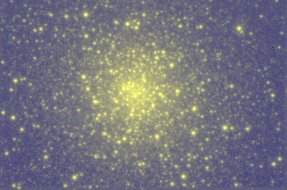There are megaliths...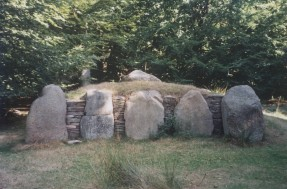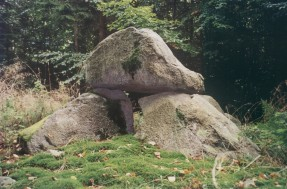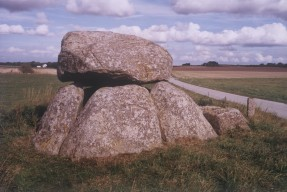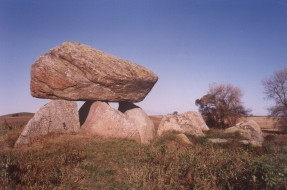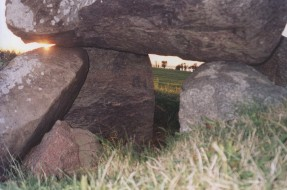And much more...

This page is intended to bring some information on the things
-- I am interested in (both scientifically and personally),
-- the students should be interested in (lectures, exercises etc.).

Since I am extremely lazy creature I shall be maintaining my www resources in
English language only. Moreover, writing in html is much too difficult.

Below I post several pictures of the partial solar eclipse occured on Jan 4, 2011.
These frames are ordered according to the time of acquisition and were taken at
09:03, 09:08, 09:18, 09:53, 10:04, and 10:17, respectively.
The eclipse started at 08:08, reached the deepest phase at 09:29, and terminated at 10:57.
(All dates are given in local time).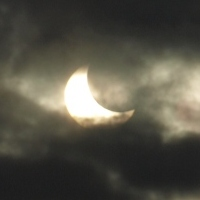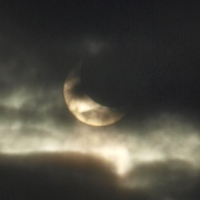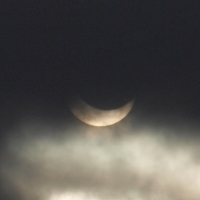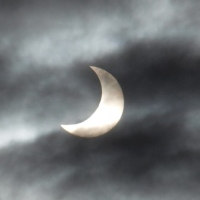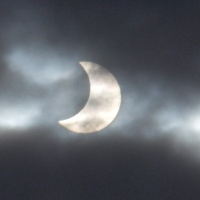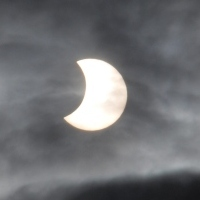Here is the manuscript of the lectures (given in summer semester of 2013) on
Pulsating stars, globular clusters and evolution of Galaxy

Here is the manuscript of my lectures in galactic astronomy (written in Polish).
It is in the PostScript format. The text is still being developed and new chapters
will be added every week or so. Students should expect frequent updates.
Current version (of Jun 7) is obligatory for all students wanting to pass examination.

Here is the first set of exercises to the lectures in galactic astronomy.
Here is the second set of exercises to the lectures in galactic astronomy.
Here is the third set of exercises to the lectures in galactic astronomy.
- Here are the data for the first exercise.
- Here are the data for the second exercise.
Here is the fourth set of exercises to the lectures in galactic astronomy.
Here is the fifth set of exercises to the lectures in galactic astronomy.
Here is the sixth set of exercises to the lectures in galactic astronomy.
Here is the seventh set of exercises to the lectures in galactic astronomy.
Here is the eighth set of exercises to the lectures in galactic astronomy.
- Here are the data for the third exercise.
Here is the ninth set of exercises to the lectures in galactic astronomy.
- Here are the data for the second exercise.
- Here are the data for the third and fourth exercises.
- Here are the data for the fifth exercise.

Here is the first set of exercises to the lectures in extragalactic astronomy.
Here is the second set of exercises to the lectures in extragalactic astronomy.
Here is the third set of exercises to the lectures in extragalactic astronomy.
Here is the fourth set of exercises to the lectures in extragalactic astronomy.
Here is the fifth set of exercises to the lectures in extragalactic astronomy.
Here is the sixth set of exercises to the lectures in extragalactic astronomy.
Here is the seventh set of exercises to the lectures in extragalactic astronomy.
Here is the eighth set of exercises to the lectures in extragalactic astronomy.

Here is the manuscript of my lectures on the programming in C (written in Polish).
It is in the PostScript format. The text is almost finished.

Here is the first set of exercises to the lectures in C programming.
Here is the second set of exercises to the lectures in C programming.
Here is the third set of exercises to the lectures in C programming.
Here is the fourth set of exercises to the lectures in C programming.
Here is the fifth set of exercises to the lectures in C programming.
Here is the sixth set of exercises to the lectures in C programming.
Here is the seventh set of exercises to the lectures in C programming.

Here is the first set of exercises to the workshop in C programming.
- Here are the data for the first exercise.
- Here are the data for the second exercise.
- Here are the data for the third exercise.
- Here are the data for the fourth exercise.
Here is the second set of exercises to the workshop in C programming.
- Here are the data for the first exercise.
- Here are the data for the second exercise.
- Here are the data for the third exercise.
- Here are the data for the fourth exercise.

Here is a gallery of the CCD pictures of different astrophysical objects taken at the
Bialkow Observatory using 0.6-meter telescope equipped with the CCD camera.

Here is the place with my resources about Danish prehistoric sites covering Late
Stone, Bronze, Iron, and Viking Ages. Right now there is only a small sample
of pictures I have taken in Danmark during the first 'observational season'
(from Aug to Dec 2003). This sample consists of 779 pictures arranged according to
the time of their aquisition. However, the full set comprises some 4000 pictures.
There is no description. It will appear in (near) future.

Here is very nice mosaic made of some pictures of Danish stone barrows. Only one
object is shown on more than one photograph. I wonder if you can identify it and
find appropriate photos?

Created and maintained by Grzegorz Kopacki. Updated on Jan 5, 2011.